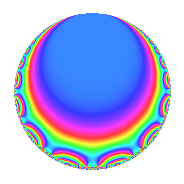# Properties

 Label 1800.1.g.cLevel 1800 Weight 1 Character orbit 1800.g Analytic conductor 0.898 Analytic rank 0 Dimension 2 Projective image $$D_{2}$$ CM/RM disc. -15, -40, 24 Inner twists 8

# Related objects

## Newspace parameters

 Level: $$N$$ = $$1800 = 2^{3} \cdot 3^{2} \cdot 5^{2}$$ Weight: $$k$$ = $$1$$ Character orbit: $$[\chi]$$ = 1800.g (of order $$2$$ and degree $$1$$)

## Newform invariants

 Self dual: No Analytic conductor: $$0.898317022739$$ Analytic rank: $$0$$ Dimension: $$2$$ Coefficient field: $$\Q(i)$$ Coefficient ring: $$\Z[a_1, a_2]$$ Coefficient ring index: $$1$$ Projective image $$D_{2}$$ Projective field Galois closure of $$\Q(\sqrt{6}, \sqrt{-10})$$ Artin image size $$16$$ Artin image $D_4:C_2$ Artin field Galois closure of 8.0.729000000.1

## $q$-expansion

The $$q$$-expansion and trace form are shown below.

 $$f(q)$$ $$=$$ $$q -i q^{2} - q^{4} + i q^{8} +O(q^{10})$$ $$q -i q^{2} - q^{4} + i q^{8} + q^{16} + 2 q^{19} -2 i q^{23} -i q^{32} -2 i q^{38} -2 q^{46} -2 i q^{47} + q^{49} + 2 i q^{53} - q^{64} -2 q^{76} + 2 i q^{92} -2 q^{94} -i q^{98} +O(q^{100})$$ $$\operatorname{Tr}(f)(q)$$ $$=$$ $$2q - 2q^{4} + O(q^{10})$$ $$2q - 2q^{4} + 2q^{16} + 4q^{19} - 4q^{46} + 2q^{49} - 2q^{64} - 4q^{76} - 4q^{94} + O(q^{100})$$

## Character Values

We give the values of $$\chi$$ on generators for $$\left(\mathbb{Z}/1800\mathbb{Z}\right)^\times$$.

 $$n$$ $$577$$ $$901$$ $$1001$$ $$1351$$ $$\chi(n)$$ $$1$$ $$-1$$ $$1$$ $$-1$$

## Embeddings

For each embedding $$\iota_m$$ of the coefficient field, the values $$\iota_m(a_n)$$ are shown below.

For more information on an embedded modular form you can click on its label.

Label $$\iota_m(\nu)$$ $$a_{2}$$ $$a_{3}$$ $$a_{4}$$ $$a_{5}$$ $$a_{6}$$ $$a_{7}$$ $$a_{8}$$ $$a_{9}$$ $$a_{10}$$
451.1
 1.00000i − 1.00000i
1.00000i 0 −1.00000 0 0 0 1.00000i 0 0
451.2 1.00000i 0 −1.00000 0 0 0 1.00000i 0 0
 $$n$$: e.g. 2-40 or 990-1000 Significant digits: Format: Complex embeddings Normalized embeddings Satake parameters Satake angles

## Inner twists

Char. orbit Parity Mult. Self Twist Proved
1.a Even 1 trivial yes
15.d Odd 1 CM by $$\Q(\sqrt{-15})$$ yes
24.f Even 1 RM by $$\Q(\sqrt{6})$$ yes
40.e Odd 1 CM by $$\Q(\sqrt{-10})$$ yes
3.b Odd 1 yes
5.b Even 1 yes
8.d Odd 1 yes
120.m Even 1 yes

## Hecke kernels

This newform can be constructed as the intersection of the kernels of the following linear operators acting on $$S_{1}^{\mathrm{new}}(1800, [\chi])$$:

 $$T_{11}$$ $$T_{17}$$